## Example Questions

### Example Question #2 : Sequences

The first term in a sequence of integers is 2 and the second term is 10. All subsequent terms are the arithmetic mean of all of the preceding terms. What is the 39th term?

5

300

1200

6

600

6

Explanation:

The first term and second term average out to 6. So the third term is 6. Now add 6 to the preceding two terms and divide by 3 to get the average of the first three terms, which is the value of the 4th term. This, too, is 6 (18/3)—all terms after the 2nd are 6, including the 39th. Thus, the answer is 6.

### Example Question #1 : Sequences

Consider the following sequence of integers:

5, 11, 23, 47

What is the 6th element in this sequence?

93

191

95

189

191

Explanation:

First, consider the change in each element.  Notice that in each case, a given element is twice the preceding one plus one:

11 = 2 * 5 + 1

23 = 11 * 2 + 1

47 = 23 * 2 + 1

To find the 6th element, continue following this:

The 5th: 47 * 2 + 1 = 95

The 6th: 95 * 2 + 1 = 191

### Example Question #2 : Sequences

The sequence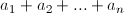begins with the numbers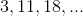and has the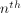term defined as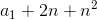, for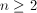.

What is the value of the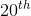term of the sequence?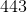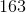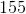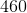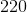Explanation:

The first term of the sequence is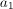, so here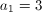, and we're interested in finding the 20th term, so we'll use n = 20.

Plugging these values into the given expression for the nth term gives us our answer.and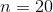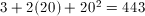### Example Question #2 : Sequences

In a sequence of numbers, the first two values are 1 and 2. Each successive integer is calculated by adding the previous two and mutliplying that result by 3. What is fifth value in this sequence?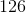Explanation:

Our sequence begins as 1, 2.

Element 3: (Element 1 + Element 2) * 3 = (1 + 2) * 3 = 3 * 3 = 9

Element 4: (Element 2 + Element 3) * 3 = (2 + 9) * 3 = 11 * 3 = 33

Element 5: (Element 3 + Element 4) * 3 = (9 + 33) * 3 = 42 * 3 = 126

### Example Question #1 : Sequences

Let Z represent a sequence of numbers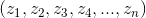wherein each term is defined as seven less than three times the preceding term.  If, what is the first term in the sequence?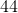Explanation:

Let us first write the value of a consecutive term in a numerical format: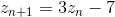Consequently,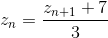Using the first equation, we can define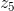in terms of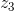: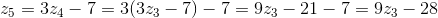This allows us to rewrite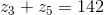as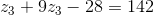Rearrangement of terms allows us to solve for: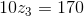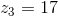Now, using our second equation, we can find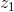, the first term: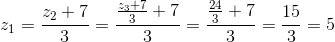### Example Question #9 : Sequences

The sequence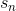is defined by: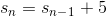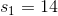What is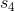?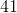Explanation:

Begin by interpreting the general definition:This means that every number in the sequence is five greater than the element preceding it.  For instance: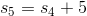It is easiest to count upwards: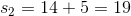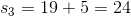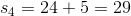### Example Question #1 : How To Find The Next Term In An Arithmetic Sequence

The sequenceis defined by: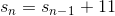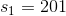What is the value of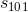?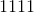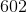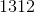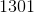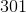Explanation:

For this problem, you definitely do not want to "count upwards" to the full value of the sequence.  Therefore, the best approach is to consider the general pattern that arises from the general definition:This means that for every element in the list, each one isgreater than the one preceding it.  For instance: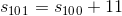Now, notice that the first element is: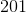The second is: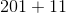The third could be represented as: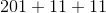And so forth...

Now, notice that for the third element, there are only two instances of.  We could rewrite our sequence: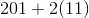This value will always "lag behind" by one.  Therefore, for the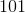st element, you will have: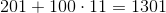### Example Question #41 : Integers

The sequenceis defined by: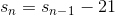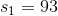What is the value of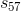?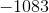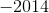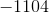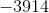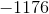Explanation:

For this problem, you definitely do not want to "count upwards" to the full value of the sequence.  Therefore, the best approach is to consider the general pattern that arises from the general definition:This means that for every element in the list, each one isless than the one preceding it.  For instance: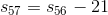Now, notice that the first element is: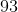The second is: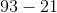The third could be represented as: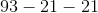And so forth...

Now, notice that for the third element, there are only two instances of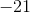.  We could rewrite our sequence: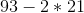This value will always "lag behind" by one.  Therefore, for the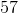th element, you will have: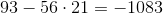### All GRE Math Resources